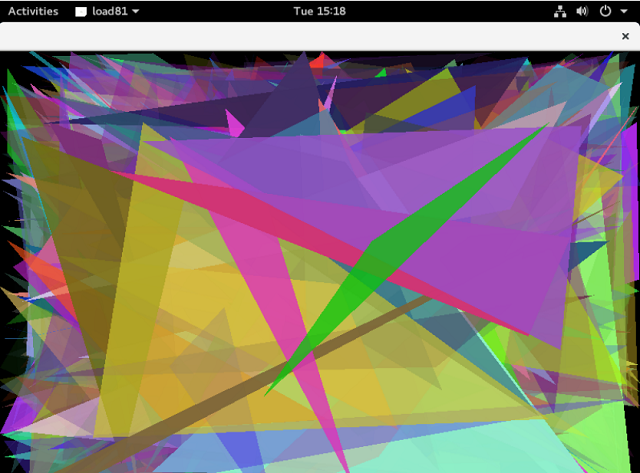## Tuesday, November 21, 2017

### Fedora 27 : load81 simple lua game engine .

Today I tested something simple with Fedora 27 and scheduling in.
This is a SDL based Lua programming environment for children called load81.

The author teel us:

The name Load81 originates from the fact that in popular Commodore home computers the command LOAD "*",8,1 would load the first program on the disk starting from the file-specified memory location.

Load81 is written in ANSI C and uses SDL and SDL_gfx and SDL_image libraries.
I installed all these libraries and everything worked very well.
Once taken from github with clones I tested the examples in the examples folder and worked well from the first time.
The examples folder come with simple examples and some simple games.
Create your lua script and use this command to run it:
``./load81 example.lua``
All running scripts require a graphical display account.
They worked very well on my normal account and I got a run-time error on my root account.
From examples, I took the triangles.lua script to illustrate how this game engine looks and works.
``````
function setup()
background(0,0,0);
end

function draw()
fill(math.random(255),math.random(255),math.random(255),math.random())
triangle(math.random(WIDTH),math.random(HEIGHT),
math.random(WIDTH),math.random(HEIGHT),
math.random(WIDTH),math.random(HEIGHT))
end``````

This is screenshot of triangles.lua script: